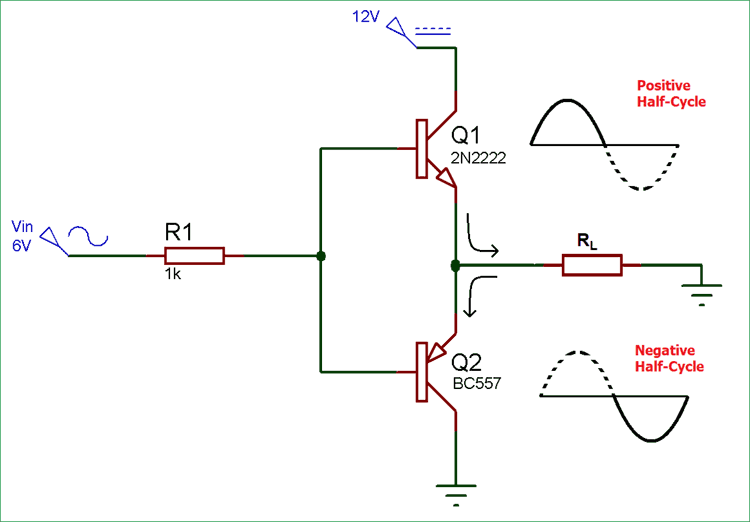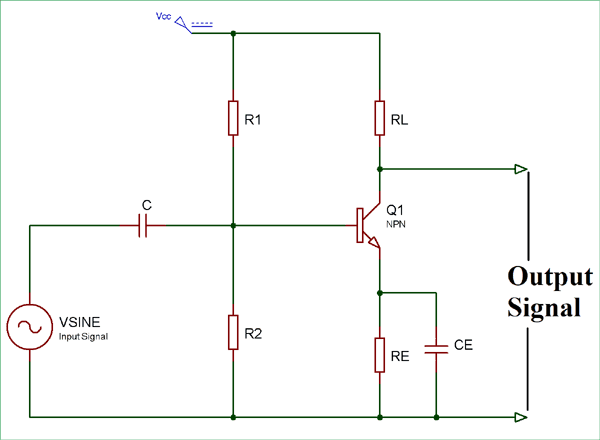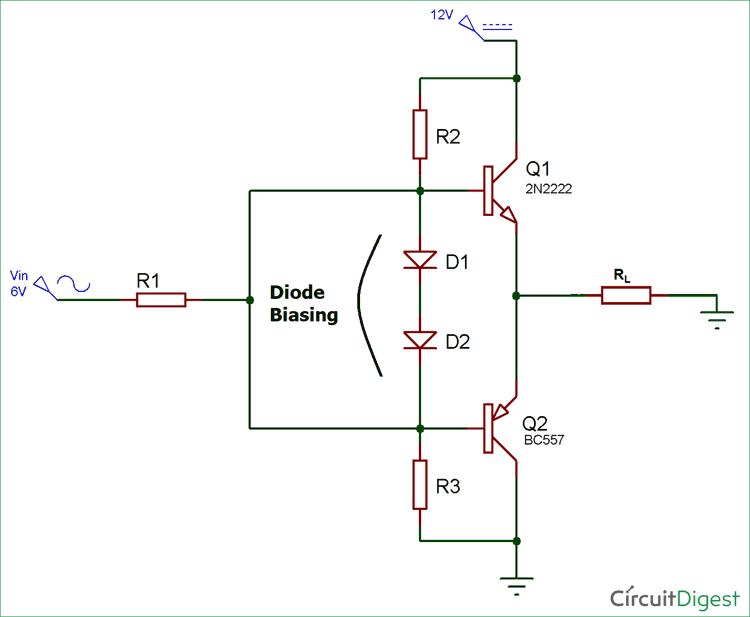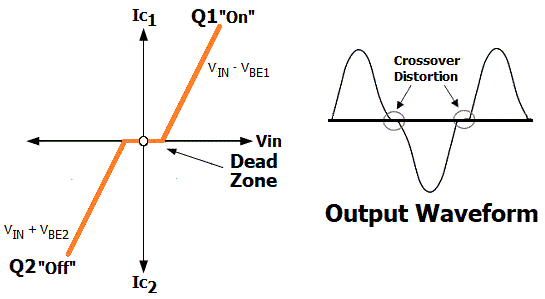# Push-Pull Amplifier Circuit

Published  August 13, 2018   1Push-Pull Amplifier is a power amplifier which is used to supply high power to the load. It consists of two transistors in which one is NPN and another is PNP. One transistor pushes the output on positive half cycle and other pulls on negative half cycle, this is why it is known as Push-Pull Amplifier. The advantage of Push-Pull amplifier is that there is no power dissipated in output transistor when signal is not present. There are three classification of Push-Pull Amplifier but generally Class B Amplifier is considered as Push Pull Amplifier.

• Class A amplifier
• Class B amplifier
• Class AB amplifier

### Class A Amplifier

Class A configuration is the most common power amplifier configuration. It consists of only one switching transistor which is set to remain ON always. It produces minimum distortion and maximum amplitude of output signal. The efficiency of Class A amplifier is very low near to 30%. The stages of the Class A amplifier allows same amount of load current to flow through it even when there is not input signal connected, therefore large heatsinks are needed for the output transistors. The circuit diagram for Class A amplifier is given below:### Class B Amplifier

Class B amplifier is the actual Push-Pull Amplifier. Efficiency of Class B amplifier is higher than Class A amplifier, as it consists of two transistors NPN and PNP. The Class B amplifier circuit is biased in such a way that each transistor will work on one half cycle of the input waveform. Therefore, the conduction angle of this type of amplifier circuit is 180 Degree. One transistor pushes the output on positive half cycle and other pulls on negative half cycle, this is why it is known as Push-Pull Amplifier. Circuit diagram for Class B amplifier is given below:Class B generally suffers from an effect known as Crossover Distortion in which signal get distorted at 0V. We know that, a transistor requires 0.7v at its base-emitter junction to turn it on. So when AC input voltage is applied to push-pull amplifier, it starts increasing from 0 and until it reaches to 0.7v, transistor remains in OFF state and we don’t get any output. Same thing happen with PNP transistor in negative half cycle of AC wave, this is called Dead Zone. To overcome this problem, diodes are used for biasing, and then the amplifier is known as Class AB Amplifier.

### Class AB Amplifier

A common method to remove that crossover distortion in Class B amplifier is to bias both the transistor at a point slightly above then the cut-off point of transistor. Then this circuit is known as Class AB amplifier circuit. Crossover distortion is later explained in this article.The Class AB amplifier circuit is the combination of both Class A and Class B amplifier. By adding the diode, transistors are biased in slightly conducting state even when no signal is present at base terminal, thus removing the crossover distortion problem.

### Materials Required

• Transformer (6-0-6)
• BC557-PNP Transistor
• 2N2222-NPN Transistor
• Resistor – 1k (2 nos)
• LED

### Working of Push-Pull Amplifier Circuit

The schematic diagram for Push-Pull amplifier circuit consists of two transistor Q1 and Q2 which are NPN and PNP respectively. When the input signal is positive Q1 starts conducting and produce a replica of the positive input at the output. At this moment Q2 remains in off condition.

Here, in this condition

`VOUT = VIN – VBE1`

Similarly, when input signal is negative Q1 turns off and Q2 starts conducting and produce a replica of the negative input at the output.

In this condition,

`VOUT = VIN + VBE2`

Now why the crossover distortion is happening when VIN reaches to zero? Let me show you rough characteristics diagram and output wave form of Push-Pull Amplifier Circuit.Transistor Q1 and Q2 cannot be simultaneously ON, for Q1 to be on we require that VIN must be greater than Vout and for Q2 Vin must be less than Vout. If VIN is equal to zero then Vout must also be equal to zero.

Now when VIN is increasing from zero, the output voltage Vout will remain zero until VIN is less than VBE1 (which is approx. 0.7v), where VBE is the voltage required to turn on the NPN transistor Q1. Hence, the output voltage is exhibiting a dead zone during the period VIN is less than VBE or 0.7v. This same thing will happen when VIN is decreasing from zero, PNP transistor Q2 won’t conduct until the VIN is greater than VBE2 (~0.7v), where VBE2 is the voltage required to turn ON transistor Q2.